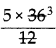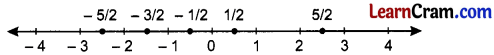# DAV Class 7 Maths Chapter 1 Worksheet 1 Solutions

The DAV Class 7 Maths Book Solutions Pdf and DAV Class 7 Maths Chapter 1 Worksheet 1 Solutions of Rational Numbers offer comprehensive answers to textbook questions.

## DAV Class 7 Maths Ch 1 WS 1 Solutions

Question 1.
Which of the following are rational numbers?
(i) -3
(ii) $$\frac{-2}{3}$$
(iii) $$\frac{4}{0}$$
(iv) $$\frac{0}{-5}$$
(i) -3 is a rational number.
(ii) $$\frac{-2}{3}$$ is a rational number.
(iii) $$\frac{4}{0}$$ is not a rational number as
(iv) $$\frac{0}{-5}$$ is a rational number.

Question 2.
Write down the rational numbers in the form $$\frac{p}{q}$$ whose numerators and denominators are given below.
(i) (-5) × 4 and -5 + 4
Numerator = (- 5) × 4 = – 20
Denominator = – 5 + 4 = – 1
Rational Number = $$\frac{-20}{-1}$$ or $$\frac{20}{1}$$ = 20

(ii) 64 ÷ 4 and 32 – 18
Numerator = 64 + 4 = 16
Denominator = 32 – 18 = 14
Rational Number = $$\frac{16}{14}=\frac{16 \div 2}{14 \div 2}=\frac{8}{7}$$Question 3.
Which of the following are positive rational numbers?
(i) $$\frac{-2}{9}$$
$$\frac{-2}{9}$$ is not positive rational number.

(ii) $$\frac{3}{-5}$$
$$\frac{3}{-5}$$ is not positive rational number.

(iii) $$\frac{4}{9}$$
$$\frac{4}{9}$$ is the positive rational number.

(iv) $$\frac{-3}{-19}$$
$$\frac{-3}{-19}$$ is the positive rational number.

(v) $$\frac{0}{-3}$$
Solution:
$$\frac{0}{-3}$$ is not positive rational number.

Question 4.
(i) Which integer is neither positive nor negative?
0 is the integer which is neither positive nor negative.

(ii) A rational number can always be written as $$\frac{p}{q}$$. Is it necessary that any number written as $$\frac{p}{q}$$ is a rational number?
No. For example, o is a rational number of the form $$\frac{p}{q}$$ where 10 and 1 are integers and q ≠ 0. But $$\frac{p}{q}$$ i.e. is not a rational number as p = 0.Question 5.
State whether the following statements are true. If not, justify your answer with an example.
(i) Every whole number is a natural number.
False; because 0 is the whole number but it is not a natural number.

(ii) Every natural number is an integer.
True, as the system of integers include all positive zero and all negative numbers.

(iii) Every integer is a whole number.
False; as whole numbers are positive whereas integers are negative and positive both, e.g. – 2 is not a whole number.

(iv) Every integer is a rational number.
True

(v) Every rational number is a fraction.
False : Fractions are never negative whereas rational numbers may be negative.
e.g. $$\frac{-2}{3}$$ is not a fraction but it is a rational number.

(vi) Every fraction is a rational number.
False, fraction cannot be negative whereas rational numbers may be negative.
e.g. $$\frac{-2}{3}$$ is a rational number but $$\frac{-2}{3}$$ is not a fraction.### DAV Class 8 Maths Chapter 1 Worksheet 1 Notes

Natural Numbers:
All positive numbers starting from 1 are called natural numbers. These numbers are used for counting.
1, 2, 3, 4, 5, 6, ………………….. are all natural numbers.

Whole Numbers:
All positive numbers including zero are called whole numbers.
0, 1, 2, 3, 4, 5 ………………….. are all whole numbers.

Integers:
All positive numbers and their corresponding negatives along with zero, constitute a system of integers.
………………….., -5, – 4, – 3, – 2, – 1, 0, 1, 2, 3, 4, 5, ………………….

Fraction:
Fraction is a part of a whole number
e.g. $$\frac{1}{4}$$, $$\frac{3}{8}$$, $$\frac{7}{5}$$ …………………..

Rational Numbers:
The numbers which are represented in the form $$\frac{a}{b}$$ where a and b are co-primes and b ≠ 0 are called rational numbers.
e.g. $$\frac{2}{3}, \frac{1}{6}, \frac{5}{7}, \frac{-3}{-4}$$ are all positive rational numbers and $$\frac{-2}{3}, \frac{1}{-6}, \frac{-11}{12}, \frac{3}{-4}$$ are all negative rational numbers.

Properties of Rational Numbers:

• If $$\frac{p}{q}$$ be a rational number and m be any integer different from zero, then $$\frac{p}{q}=\frac{p \times m}{q \times m}$$
• If $$\frac{p}{q}$$ be a rational number and m be a common divisor of p and q, then $$\frac{p}{q}=\frac{p \div m}{q \div m}$$
• Two rational numbers $$\frac{p}{q}$$ and $$\frac{r}{s}$$ are said to be equivalent (equal) if p × s = q × r.

Standard form of a Rational Number:
A rational number $$\frac{p}{q}$$ is said to be in standard form if q is positive and HCF of p and q is 1.

Absolute Value of a Rational Number:

• Absolute value of every rational number other than zero is positive.
• The absolute value of zero is zero itself.
• Absolute value of a rational number is greater than or equal to the number itself.

Comparing Rational Numbers:

(i) By representing on number line:
Rational numbers can be compared easily when they are represented on the number line. Any number on the number line is greater than any other number lying to the left of it. Any number on a number line is less than any other number lying to the right of it.

(ii) Without representing on number line:
If and are two rational numbers with q and s positive integers then
if $$\frac{p}{q}>\frac{r}{s}$$ if p × s > q × r,
$$\frac{p}{q}<\frac{r}{s}$$ if p × s < q × r and
$$\frac{p}{q}=\frac{r}{s}$$ if p × s = q × rExample 1:
Which of the following are positive rational numbers?
(i) $$\frac{-1}{-2}$$
(ii) $$\frac{5}{-6}$$
(iii) $$\frac{2}{3}$$
(iv) $$\frac{-4}{7}$$
(i) $$\frac{-1}{-2}$$ is a positive rational number as the numerator and the denominator have the same sign.
(ii) $$\frac{5}{-6}$$ is not a positive rational number as the sign of the numerator and the denominator are different.
(ii) $$\frac{2}{3}$$ is a positive rational number.
(iv) $$\frac{-4}{7}$$ is not a positive rational number.

Example 2:
Show that $$\frac{-2}{7}$$ and $$\frac{6}{-21}$$ are equivalent rational numbers.
(- 2) × (- 21) = 6 × 7
42 = 42
Hence, $$\frac{-2}{7}$$ and $$\frac{6}{-21}$$ are equivalent rational numbers.

Example 3:
Write two rational numbers which are equivalent to $$\frac{2}{3}$$.
Multiplying the numerator and the denominator of the given fraction by the same number, we get
$$\frac{2}{3}=\frac{2 \times 2}{3 \times 2}=\frac{4}{6}$$,
$$\frac{2}{3} \times \frac{3}{3}=\frac{6}{9}$$
Hence, the required rational numbers are $$\frac{4}{6}$$ and $$\frac{6}{9}$$.Example 4:
Express $$\frac{24}{-54}$$ in the standard form.
HCF of 24 and 54 is 6
∴ Dividing the numerator and denominator by 6, we get
$$\frac{24 \div 6}{-54 \div 6}=\frac{4}{-9}$$
Hence, the simplest standard form $$\frac{4}{-9}$$

Example 5:
Find the value of x such that the given rational numbers become equivalent.
$$\frac{x}{36}=\frac{5}{12}$$.
$$\frac{x}{36}=\frac{5}{12}$$ will be equivalent if
x × 12 = 5 × 36
⇒ x == 15
Hence, x = 15

Example 6.
What is absolute value of a rational number? Find the absolute values of the given rational numbers.
$$\frac{-2}{3}, \frac{6}{-7}, \frac{2}{3}$$, – 4, 0
The absolute value of a rational number is its numerical value without its sign.
Here, absolute values of the given rational numbers are
$$\left|\frac{-2}{3}\right|=\frac{2}{3},\left|\frac{6}{-7}\right|=\frac{6}{7},\left|\frac{2}{3}\right|=\frac{2}{3},|-4|=4,|0|=0$$.

Example 7:
Represent the following rational numbers on number line.
$$\frac{-3}{2}, \frac{1}{2}, \frac{-1}{2}, \frac{5}{2}, \frac{-5}{2}$$Example 8:
Compare $$\frac{2}{3}$$ and $$\frac{3}{5}$$.
$$\frac{2}{3} \times \frac{5}{5}=\frac{10}{15}$$
and $$\frac{3}{5} \times \frac{3}{3}=\frac{9}{15}$$
∴ $$\frac{2}{3}>\frac{3}{5}$$.